# Some simple visual comparisons of model selection techniques

The goal of model selection is to find a model that provides the best fit to a set of data, without overfitting the data. Different criterion for assessing the degree of overfitting abound; typically they make reference to the number of parameters a model includes. Too few parameters, and your model will not be flexible enough to fit the data. Too many, and your model will be too flexible and end up overfitting the data.

I made a little program that calculates and plots different measures of model quality as a function of the number of parameters in the model, for any choice of true distribution. The models used in this program are all just polynomial fits; the kth model is the set of all (k-1)-order polynomials. I’ll show off some of the resulting plots here!

***

True distribution: y(x) = x2

10 data points100 data points1000 data points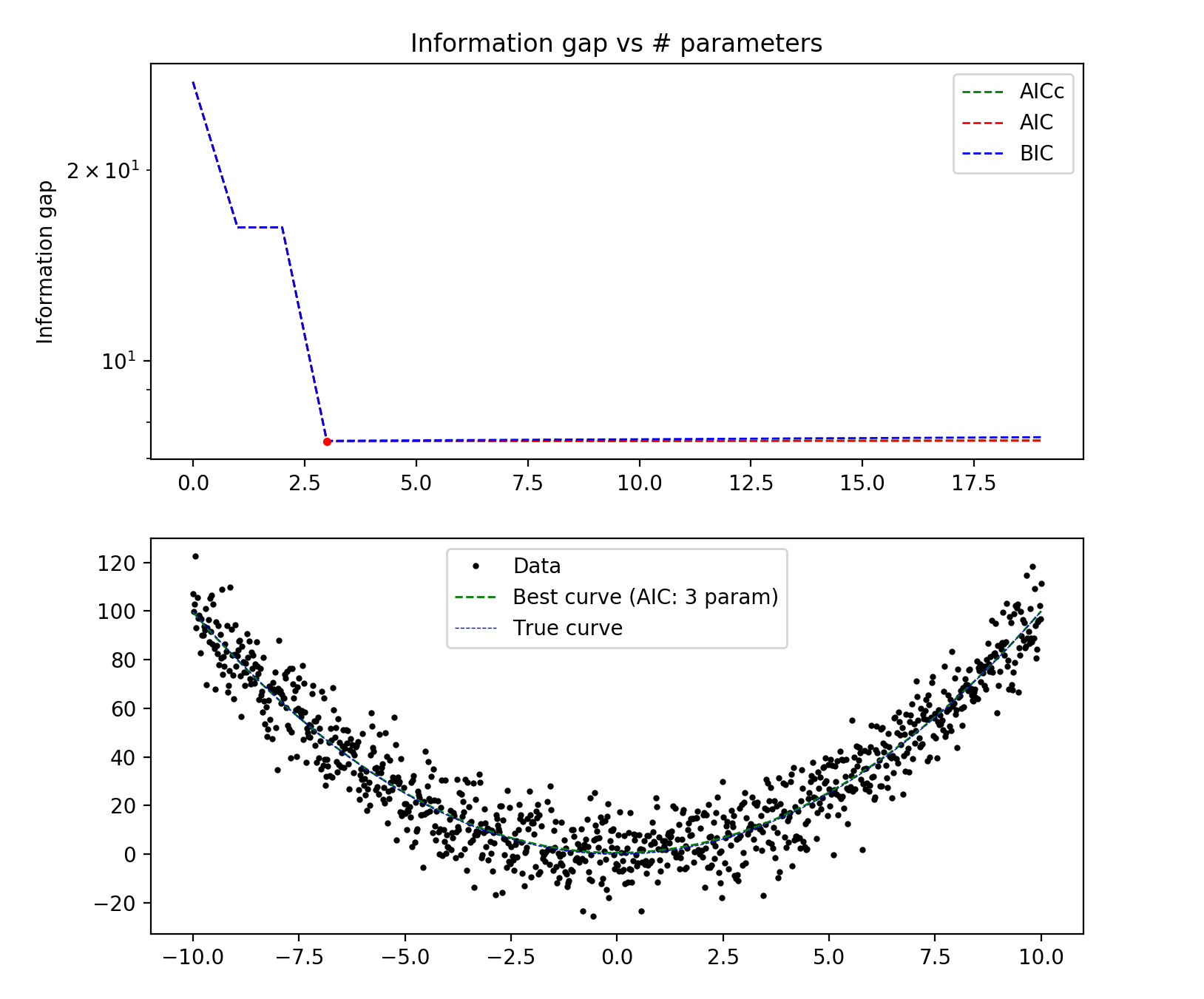Some things to notice:

• All three of BIC, AIC, and AICc give the same (and correct) answer, even for a data set of only 10 points.
• The difference between AICc and AIC becomes pretty much irrelevant for large enough data sets.
• BIC always penalizes complexity more than AIC
• The complexity penalty is pretty nearly matched by the improvement in fit for large numbers of parameters, but slightly outweighs it.

True distribution: y = x3/10 + x2 – 10x

10 data points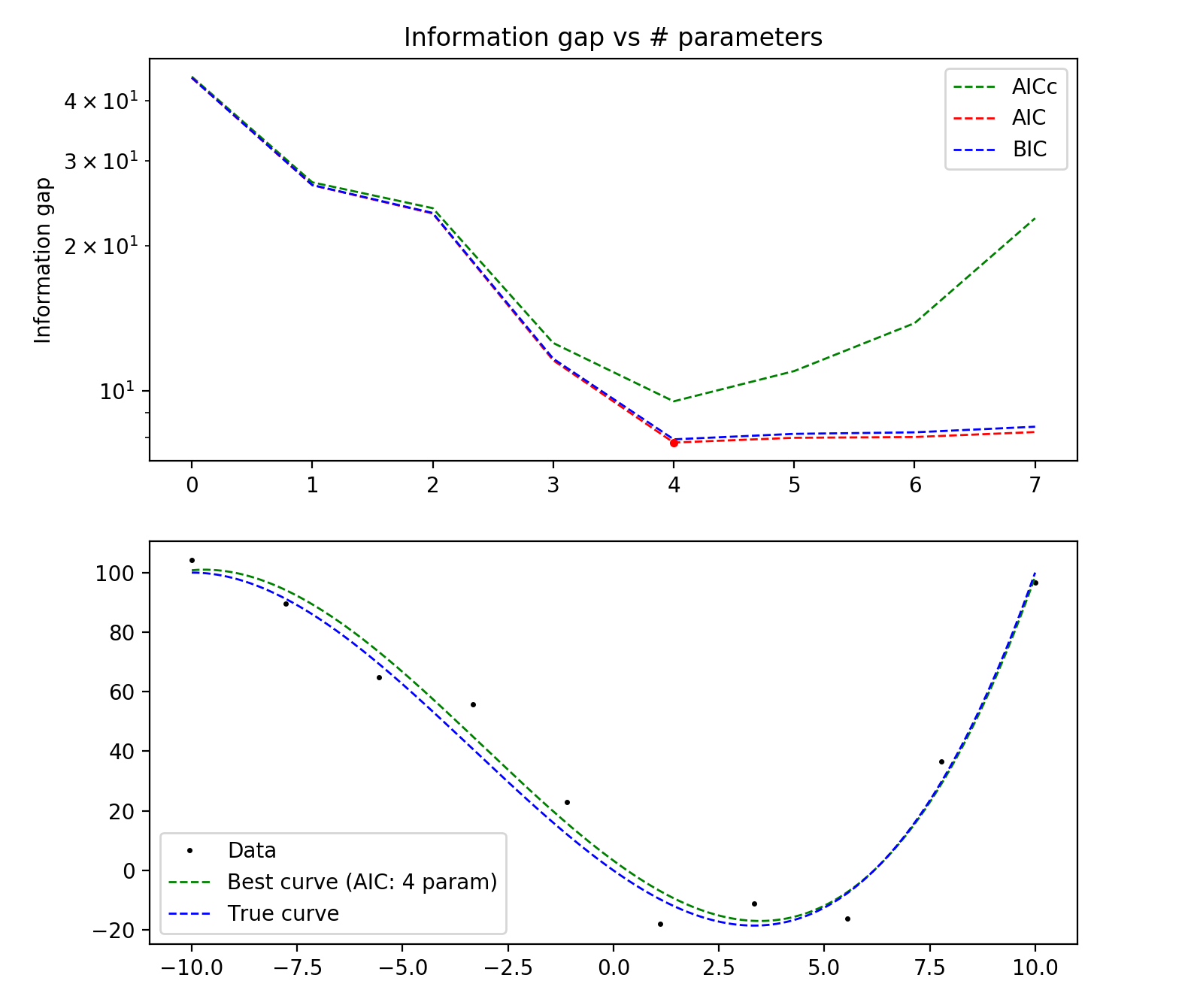100 data points1000 data points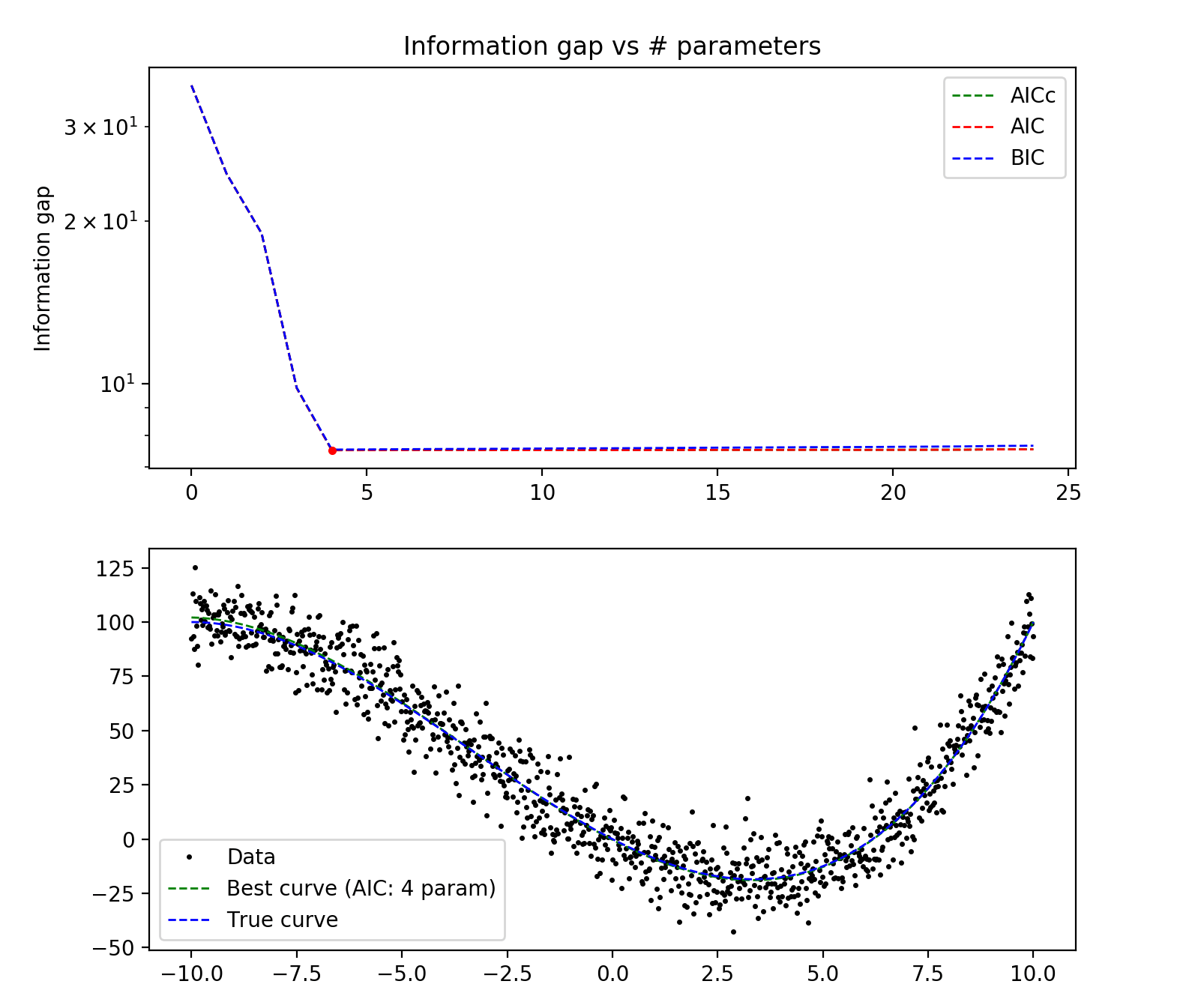Now let’s look at an example where the true distribution is not actually in any of the models.

True distribution: y = e-x/2

20 data points100 data points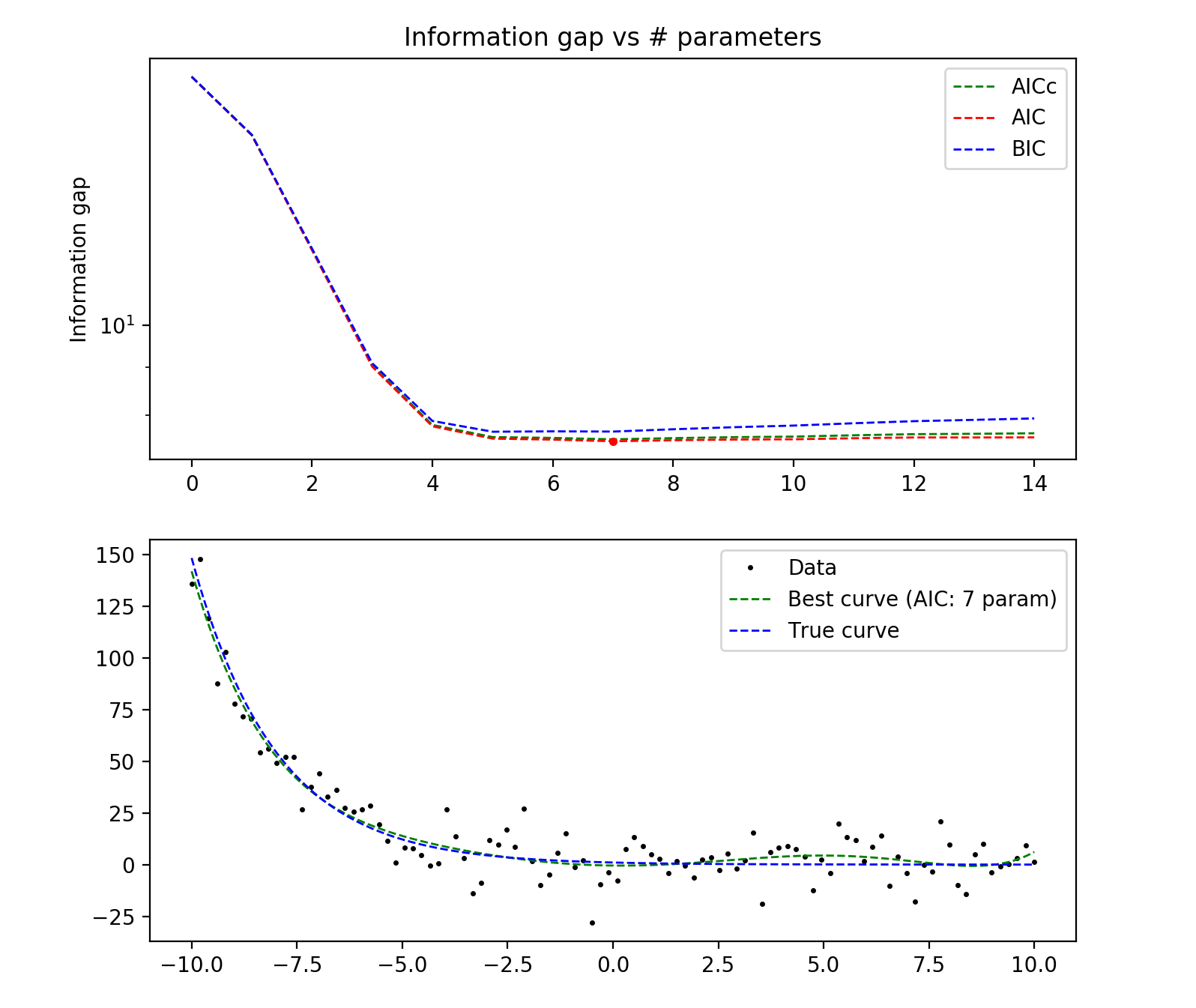1000 data pointsHere we begin to see some disagreement between the different methods! For N=20, AICc would have recommended the optimal model as k = 4 (a third order polynomial), while AIC and BIC both recommended k = 5. In addition, we see that the same method gives different answers as the number of data points rises (5 to 7 to 6 parameters)

Regardless, we still see that all three methods succeed in preventing overfitting, and do a fairly good job at catching the underlying trend in the data. However, the question of which model is optimal becomes a little more ambiguous.

One final example, which we’ll make especially difficult for a polynomial model:

True distribution: y = 10*sin(x)

N = 20N = 100N = 1000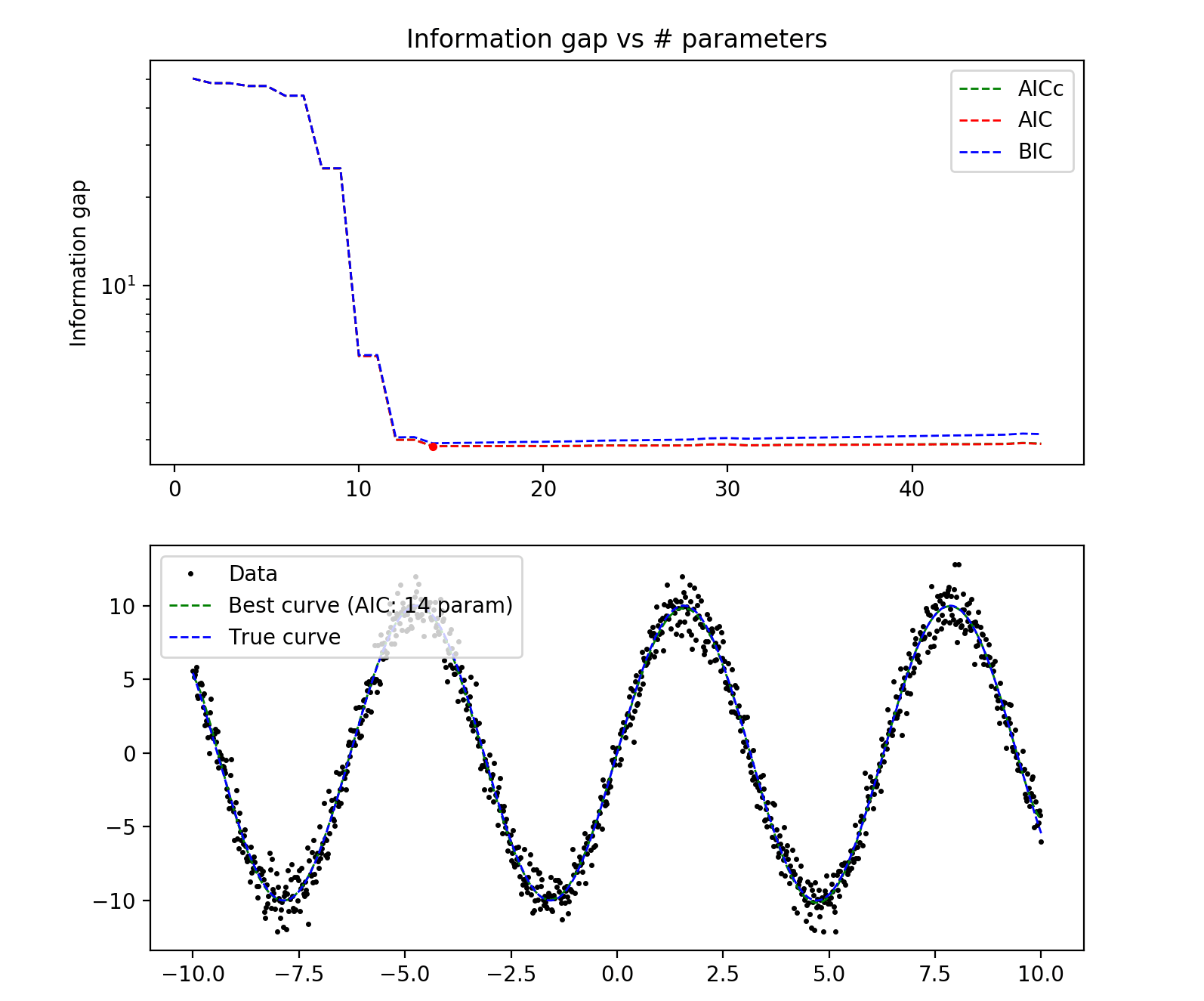Again we see that all of the model selection criterion give similar answers, and the curves generated nicely align with the true curve. It looks like 11 to 13 order polynomials do a good job at modeling a sine wave on this scale.

It’s interesting to watch the jagged descent of the criteria as you approach the optimal number of parameters from below. For some reason, it looks like adding a single extra parameter is generally unhelpful for this problem, but adding two is helpful. I suspect that this is related to the fact that sin(x) is an odd function, so adding an even function with a tweakable parameter out front doesn’t do much for your model fit.

By the end, we see the optimal curve beautifully aligning with the true curve, not getting distracted by the noise in the data. Seeing these plots helps give a bit of an intuition about how different techniques penalize complexity and reward goodness of fit to data. I want to eventually add cross validation scores in to these plots as well, to see how they compare to the others.